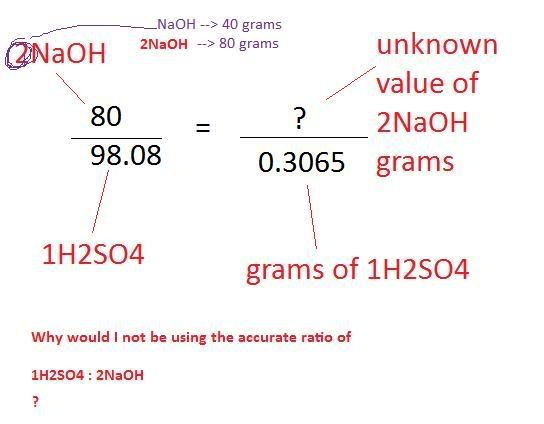December 11, 2023, 04:37:34 PM
Forum Rules: Read This Before Posting

###Topic: Stoichiometry Problem  (Read 7656 times)

0 Members and 1 Guest are viewing this topic.

####positiveion

• Regular Member
•• Posts: 79
• Mole Snacks: +2/-7##### Stoichiometry Problem
« on: October 24, 2009, 12:34:34 AM »
Sulfuric acid and sodium hydroxide react together according to the equation:

H2SO4 + 2NaOH --> Na2SO4 + 2H2O

What volume of 0.250 mol dm^-3 NaOH is required to neutralise exactly 25.0 cm^3 of 0.125 mol dm^-3 H2SO4?

How I've been doing it

H2SO4:
molarity --> 98.08
no. moles of h2so4--> 0.003125
no. grams of h2s04--> 0.3065

2NaOH:
molarity --> 80
no. moles --> ?
no. grams--> ?

Finding no. grams in 2NaOH:
80/98.08 = ?/0.3065
? = 0.25

Determining how many moles that is:
0.25/80
= 0.003125 moles

Determining how much that is out of 0.250 which is the no. moles per litre for the NaOH:
0.250 moles / 0.003125 = 80

Therefore:
1/80
=0.0125 cm^3

Which is wrong.

The actual answer is 25 cm^3

####UG

• Full Member
•• Posts: 822
• Mole Snacks: +134/-15
• Gender:##### Re: Stoichiometry Problem
« Reply #1 on: October 24, 2009, 12:39:49 AM »
You're on the right track there, you've just forgotten the ratio, which is one acid molecule for every TWO base molecules. So whatever you get for the amount of moles of sulfuric acid, the number of moles of NaOH will be doubled. You should get the correct answer now.

####positiveion

• Regular Member
•• Posts: 79
• Mole Snacks: +2/-7##### Re: Stoichiometry Problem
« Reply #2 on: October 24, 2009, 12:47:44 AM »
1). I don't really understand that because I think I did remember. In this following ratio thing:

80/98.08 = ?/0.3065
? = 0.25

80 is not the molarity of NaOH, its the molarity of 2NaOH -- am i not doing what you said?

2). I tried this in a different way. So first the no. moles in H2SOr is 0.003125. I multiply that by 2, and get 0.00625.

0.250/0.00625 = 40

therefore, 1/40 = 0.025

So it should be 0.025 cm^3, right? That's still wrong.

####UG

• Full Member
•• Posts: 822
• Mole Snacks: +134/-15
• Gender:##### Re: Stoichiometry Problem
« Reply #3 on: October 24, 2009, 12:51:09 AM »
I'm not sure I follow your working but the answer 0.025 would be litres, which is 25 mL, which is 25cm3

####positiveion

• Regular Member
•• Posts: 79
• Mole Snacks: +2/-7##### Re: Stoichiometry Problem
« Reply #4 on: October 24, 2009, 12:58:25 AM »
OHH okay but can i still clarify what i did wrong in the first way?

just copied and pasted from before
H2SO4:
molarity --> 98.08
no. moles of h2so4--> 0.003125
no. grams of h2s04--> 0.3065

2NaOH:
molarity --> 80
no. moles --> ?
no. grams--> ?

Finding no. grams in 2NaOH:
80/98.08 = ?/0.3065
? = 0.25

^ in this above thing, the ratio I am attempting to solve is

no. grams in 1 mole (i.e. no grams as directly translated from amu) for 2naoh/ no. grams in 1 mole (i.e. no grams as directly translated from amu) for h2so4
= no. grams given to us in this problem for 2naoh/ no. grams given to us in this problem for h2so4

therefore:

80/98.09 = ?/0.3065

What I was saying just now, is that

NaOH:
Na= 22.99
O= 16
H=1.01

But because the equation said 2NaOH I multiplied that by 2 to make 80

Whereas for H2SO4
H= 2*1.01
S=32.06
O=16*4

Therefore when I do

80/98.08 = ?/0.3065

Isn't that already including the accurate ratio in my problem solving?

####UG

• Full Member
•• Posts: 822
• Mole Snacks: +134/-15
• Gender:##### Re: Stoichiometry Problem
« Reply #5 on: October 24, 2009, 01:07:27 AM »
I find your working way too complicated, but that's probably just teaching difference, I only used one formula n(moles) = c(Concentration) x V(Volume). You don't need to work out the molarity and the number of grams, cause you don't need to. n(sulfuric acid) = cV = 0.025(volume in litres) x 0.125 (conc.) = 0.003125 moles. So n(NaOH) = 0.00625 moles, therefore V(NaOH) = n/C = 0.00625/0.25 = 0.025 L = 25 mL = 25cm3. Really, this way is much faster and easier.

####positiveion

• Regular Member
•• Posts: 79
• Mole Snacks: +2/-7##### Re: Stoichiometry Problem
« Reply #6 on: October 24, 2009, 01:21:11 AM »
No I understand what you mean and I'm glad this simpler way works, I just really want to know WHY my previous way doesn't work.

Hm let me try to explain again in a less complicated way.

NaOH:
Na= 22.99
O= 16
H=1.01
2NaOH = 40*2 = 80

H2SO4
H= 2*1.01
S=32.06
O=16*4####UG

• Full Member
•• Posts: 822
• Mole Snacks: +134/-15
• Gender:##### Re: Stoichiometry Problem
« Reply #7 on: October 24, 2009, 01:26:39 AM »
Okay I see, so for the number of grams of NaOH you got 0.25 grams right? Yeah, well you gotta work out how many moles that is = 0.25/40 = 0.00625 moles, so then the volume you need is calculated by n/C = 0.00625/0.25 = 0.025 L = 25cm3

####positiveion

• Regular Member
•• Posts: 79
• Mole Snacks: +2/-7##### Re: Stoichiometry Problem
« Reply #8 on: October 24, 2009, 01:31:04 AM »
OHHH okay I got it now.

THANK YOU!!

####UG

• Full Member
•• Posts: 822
• Mole Snacks: +134/-15
• Gender:##### Re: Stoichiometry Problem
« Reply #9 on: October 24, 2009, 01:32:48 AM »
No problem, any time